# Class 8 RD Sharma Solutions – Chapter 17 Understanding Shapes Special Types Of Quadrilaterals – Exercise 17.1 | Set 1

• Last Updated : 16 Apr, 2021

### Question 1. Given below is a parallelogram ABCD. Complete each statement along with the definition or property used.

(ii) ∠DCB =

Attention reader! All those who say programming isn't for kids, just haven't met the right mentors yet. Join the  Demo Class for First Step to Coding Coursespecifically designed for students of class 8 to 12.

The students will get to learn more about the world of programming in these free classes which will definitely help them in making a wise career choice in the future.

(iii) OC =

(iv) ∠DAB + ∠CDA =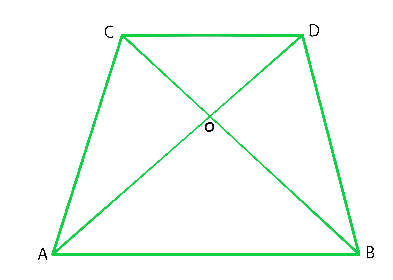Solution:

(i) AD = BC. Because, diagonals bisect each other in a parallelogram.

(ii) ∠DCB = ∠BAD. Because, alternate interior angles are equal.

(iii) OC = OA. Because, diagonals bisect each other in a parallelogram.

(iv) ∠DAB+ ∠CDA = 180°. Because sum of adjacent angles in a parallelogram is 180°.

### Question 2. The following figures are parallelograms. Find the degree values of the unknowns x, y, z.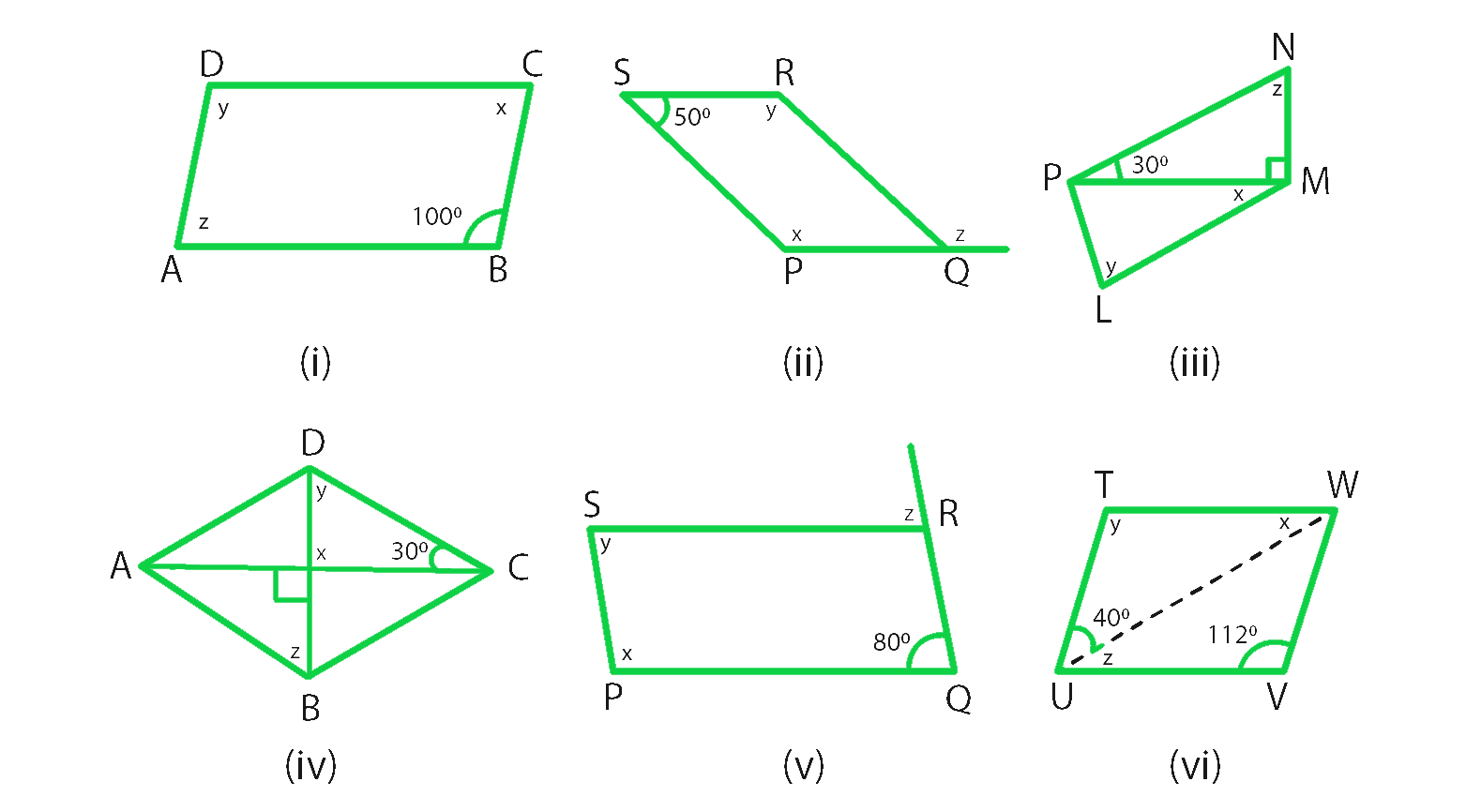Solution:

(i) From figure we conclude that,

∠ABC = ∠y = 100o (Opposite angles are equal in a parallelogram)

∠x + ∠y = 180o (sum of adjacent angles is = 180° in a parallelogram)

∠x + 100° = 180°

∠x = 180° – 100° = 80°

Hence, ∠x = 80° ∠y = 100° ∠z = 80° (opposite angles are equal in a parallelogram)

(ii) From figure we conclude that,

∠RSP + ∠y = 180° (sum of adjacent angles is = 180° in a parallelogram)

∠y + 50° = 180°

∠y = 180° – 50° = 130°

Hence, ∠x = ∠y = 130° (opposite angles are equal in a parallelogram)

From figure, we conclude that,

∠RSP = ∠RQP = 50° (opposite angles are equal in a parallelogram)

∠RQP + ∠z = 180° (linear pair)

50° + ∠z = 180°

∠z = 180° – 50° = 130°

Hence, ∠x = 130°, ∠y = 130° and ∠z = 130°.

(iii) As we know that,

In ΔPMN ∠NPM + ∠NMP + ∠MNP = 180° (Sum of all the angles of a triangle is 180°)

30° + 90° + ∠z = 180°

∠z = 180°-120° = 60°

From figure, we conclude that,

∠y = ∠z = 60° (opposite angles are equal in a parallelogram)

∠z = 180°-120° (sum of the adjacent angles is equal to 180° in a parallelogram)

∠z = 60°

∠z + ∠LMN = 180° (sum of the adjacent angles is equal to 180° in a parallelogram)

60° + 90°+ ∠x = 180°

∠x = 180°-150° = 30°

Hence, ∠x = 30° ∠y = 60° ∠z = 60°

(iv) From figure we conclude that,

∠x = 90° [vertically opposite angles are equal]

In ΔDOC, ∠x + ∠y + 30° = 180° (Sum of all the angles of a triangle is 180°)

90° + 30° + ∠y = 180°

∠y = 180°-120°

∠y = 60°

∠y = ∠z = 60° (alternate interior angles are equal)

Hence, ∠x = 90° ∠y = 60° ∠z = 60°

(v) From figure we conclude that,

∠x + ∠POR = 180° (sum of the adjacent angles is equal to 180° in a parallelogram)

∠x + 80° = 180°

∠x = 180°-80° = 100°

∠y = 80° (opposite angles are equal in a parallelogram)

∠SRQ =∠x = 100°

∠SRQ + ∠z = 180° (Linear pair)

100° + ∠z = 180°

∠z = 180°-100° = 80°

Hence, ∠x = 100°, ∠y = 80° and ∠z = 80°.

(vi) From figure we conclude that,

∠y = 112° (In a parallelogram opposite angles are equal)

∠y + ∠VUT = 180° (In a parallelogram sum of the adjacent angles is equal to 180°)

∠z + 40° + 112° = 180°

∠z = 180°-152° = 28°

∠z =∠x = 28° (alternate interior angles are equal)

Hence, ∠x = 28°, ∠y = 112°, ∠z = 28°.

### Question 3. Can the following figures be parallelograms? Justify your answer.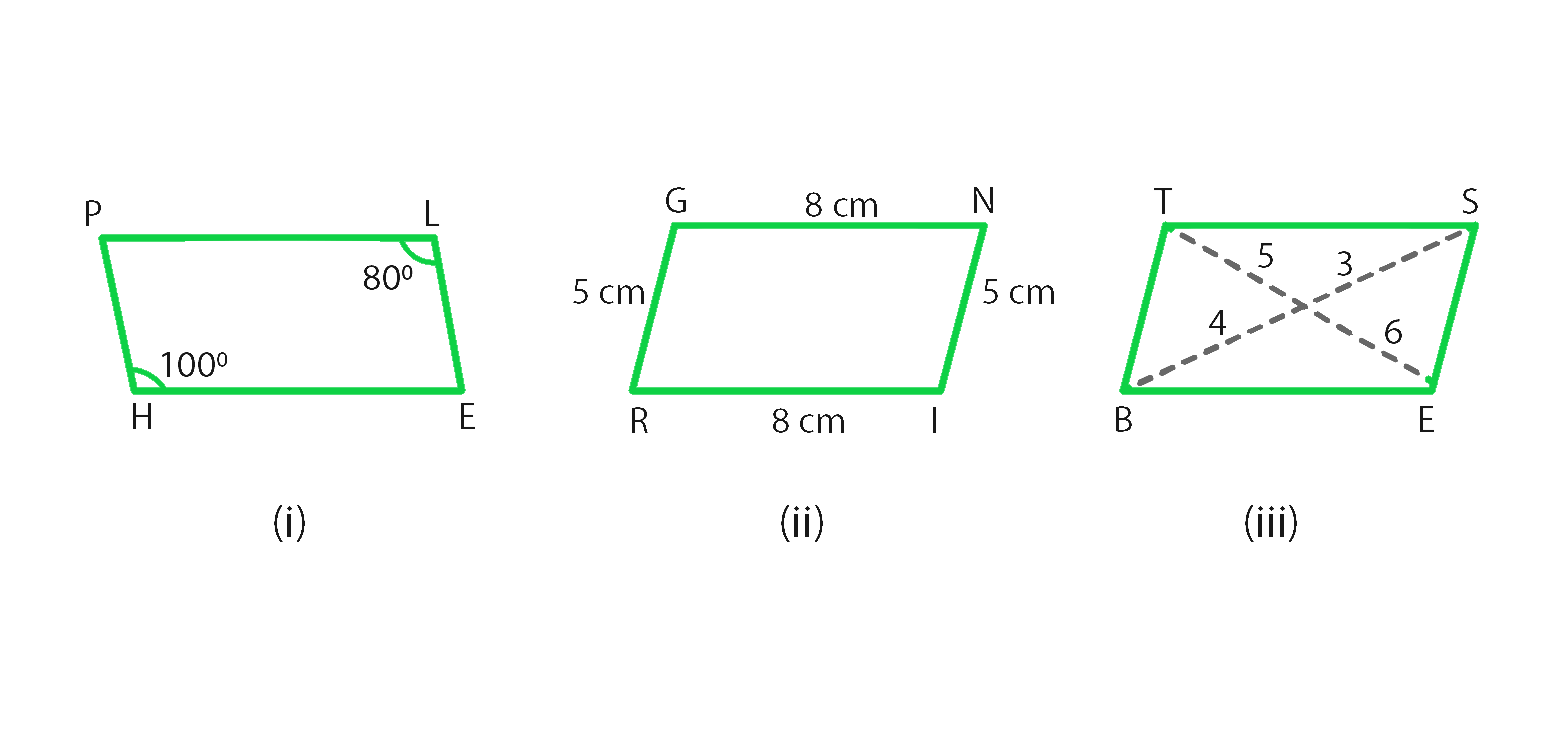Solution:

(i) No, as we know that opposite angles are equal in a parallelogram.

(ii) Yes, as we know that opposite sides are equal and parallel in a parallelogram.

(iii) No, as we know that the diagonals bisect each other in a parallelogram.

### Question 4. In the adjacent figure HOPE is a parallelogram. Find the angle measures x, y, and z. State the geometrical truths you use to find them.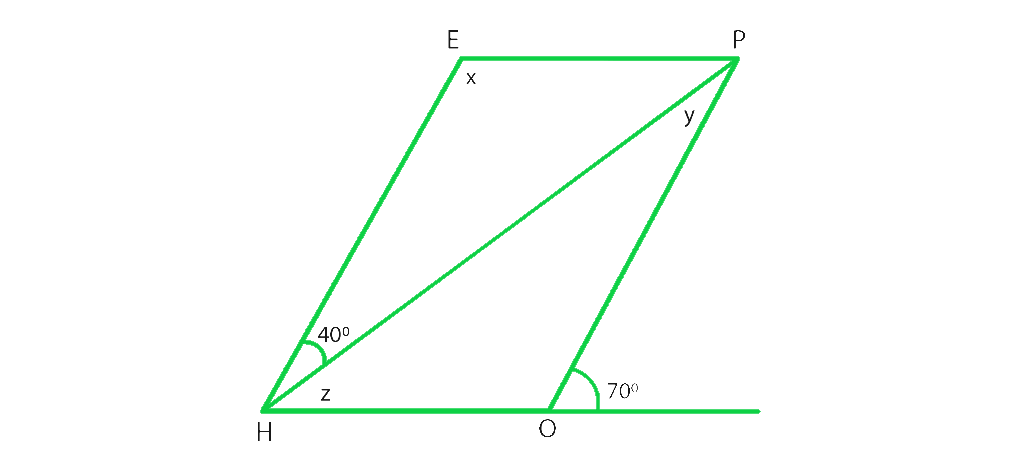Solution:

As we know that,

∠POH + 70° = 180° (Linear pair)

∠POH = 180°-70° = 110°

∠POH = ∠x = 110° (opposite angles are equal in a parallelogram)

∠x + ∠z + 40° = 180° (sum of the adjacent angles is equal to 180° in a parallelogram)

110° + ∠z + 40° = 180°

∠z = 180° – 150° = 30°

∠z +∠y = 70°

∠y + 30° = 70°

∠y = 70°- 30° = 40°

### Question 5. In the following figures, GUNS and RUNS are parallelograms. Find x and y.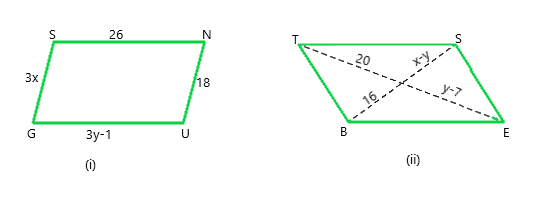Solution:

From figure, we conclude that,

(i) 3y – 1 = 26 (opposite sides are of equal length in a parallelogram)

3y = 26 + 1

y = 27/3 = 9

3x = 18 (opposite sides are of equal length in a parallelogram)

x = 18/3= 6

Hence, x = 6 and y = 9

(ii) y – 7 = 20 (diagonals bisect each other in a parallelogram)

y = 20 + 7 = 27

x – y = 16 (diagonals bisect each other in a parallelogram)

x -27 = 16

x = 16 + 27 = 43

Hence, x = 43 and y = 27

### Question 6. In the following figure RISK and CLUE are parallelograms. Find the measure of x.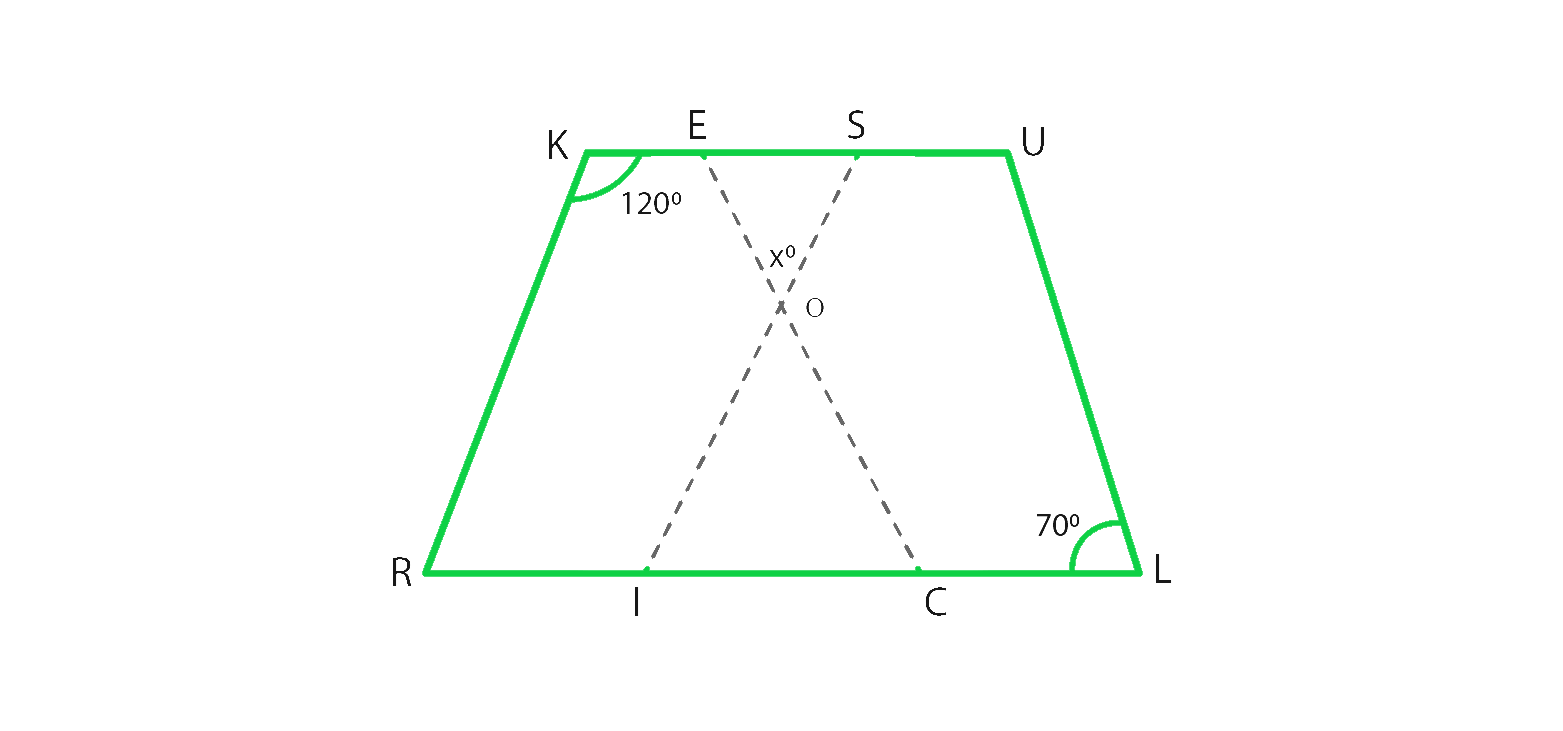Solution:

From figure, we conclude that,

In parallelogram RISK

∠RKS + ∠KSI = 180° (sum of the adjacent angles is equal to 180° in a parallelogram)

120° + ∠KSI = 180°

∠KSI = 180° – 120° = 60°

In parallelogram CLUE,

∠CEU = ∠CLU = 70° (opposite angles are equal in a parallelogram)

In ΔEOS,

70° + ∠x + 60° = 180° (Sum of angles of a triangles is 180°)

∠x = 180° – 130° = 50°

Hence, x = 50°

### Question 7. Two opposite angles of a parallelogram are (3x – 2)° and (50 – x)°. Find the measure of each angle of the parallelogram.

Solution:

As we know that the opposite angles of a parallelogram are equal.

So, (3x – 2)° = (50 – x)°

3xo – 2° = 50° – x°

3x° + xo = 50° + 2°

4x° = 52°

xo = 52°/4 = 13°

The opposite angles are,

(3x – 2)° = 3×13 – 2 = 37°

(50 – x)° = 50 – 13 = 37°

As we know that Sum of adjacent angles = 180°

Other two angles are 180° – 37° = 143°

Hence, Measure of each angle is 37°, 143°, 37°, 143°.

### Question 8. If an angle of a parallelogram is two-third of its adjacent angle, find the angles of the parallelogram.

Solution:

Let us assume that one of the adjacent angle as x°,

then other adjacent angle is = 2x°/3

As we know that sum of adjacent angles = 180°

Therefore,

x° + 2x°/3 = 180°

(3x° + 2x°)/3 = 180°

5x°/3 = 180°

x° = 180°×3/5 = 108°

Other angle is = 180° – 108° = 72°

Hence, Angles of a parallelogram are 72°, 72°, 108°, 108°.

### Question 9. The measure of one angle of a parallelogram is 70°. What are the measures of the remaining angles?

Solution:

Let us assume that the one of the adjacent angle as x°

As we know that sum of adjacent angles = 180°

Therefore,

x° + 70° = 180°

x° = 180° – 70° = 110°

Hence, Measures of the remaining angles are 70°, 70°, 110° and 110°

### Question 10. Two adjacent angles of a parallelogram are as 1: 2. Find the measures of all the angles of the parallelogram.

Solution:

Let us assume that one of the adjacent angle as x°,

then other adjacent angle = 2x°

As we know that sum of adjacent angles = 180°

Therefore,

x° + 2x° = 180°

3x° = 180°

x° = 180°/3 = 60°

So other angle is 2x = 2×60 = 120°

Hence, Measures of the remaining angles are 60°, 60°, 120° and 120°

### Question 11. In a parallelogram ABCD, ∠D= 135°, determine the measure of ∠A and ∠B.

Solution:

Given that,

one of the adjacent angle ∠D = 135°

Let us assume that other adjacent angle ∠A be = x°

As we know that sum of adjacent angles = 180°

x° + 135° = 180°

x° = 180° – 135° = 45°

∠A = x° = 45°

As we know that the opposite angles are equal in a parallelogram.

Therefore, ∠A = ∠C = 45°

and ∠D = ∠B = 135°.

### Question 12. ABCD is a parallelogram in which ∠A = 70°. Compute ∠B, ∠C, and ∠D.

Solution:

Given that,

one of the adjacent angle ∠A = 70°

and other adjacent angle ∠B is = x°

As we know that sum of adjacent angles = 180°

x° + 70° = 180°

x° = 180° – 70° = 110°

∠B = x° = 110°

As we know that the opposite angles are equal in a parallelogram.

Therefore, ∠A = ∠C = 70°

and ∠D = ∠B = 110°

### Question 13. The sum of two opposite angles of a parallelogram is 130°. Find all the angles of the parallelogram.

Solution:

From figure, we conclude that, ABCD is a parallelogram

∠A + ∠C = 130°

Here ∠A and ∠C are opposite angles

Therefore ∠C = 130/2 = 65°

As we know that sum of adjacent angles is 180

∠B + ∠D = 180

65 + ∠D = 180

∠D = 180 – 65 = 115

∠D = ∠B = 115 (Opposite angles)

Hence, ∠A = 65°, ∠B = 115°, ∠C = 65° and ∠D = 115°.

### Question 14. All the angles of a quadrilateral are equal to each other. Find the measure of each. Is the quadrilateral a parallelogram? What special type of parallelogram is it?

Solution:

Let us assume that each angle of a parallelogram as xo

As we know that sum of angles = 360°

x° + x° + x° + x° = 360°

4 x° = 360°

x° = 360°/4 = 90°

Hence, each angle is 90°

Yes, this quadrilateral is a parallelogram.

Since each angle of a parallelogram is equal to 90°, so it is a rectangle.

### Question 15. Two adjacent sides of a parallelogram are 4 cm and 3 cm respectively. Find its perimeter.

Solution:

As we know that opposite sides of a parallelogram are parallel and equal.

therefore, Perimeter = Sum of all sides (there are 4 sides)

Perimeter = 4 + 3 + 4 + 3 = 14 cm

Hence, Perimeter is 14 cm.

### Chapter 17 Understanding Shapes Special Types Of Quadrilaterals – Exercise 17.1 | Set 2

My Personal Notes arrow_drop_up